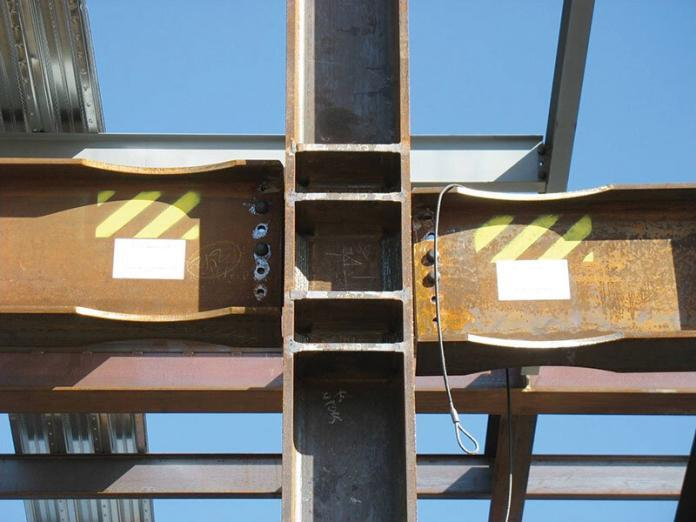# Analysis and Properties of Plastic SectionsIn structural engineering designs, plastic section properties are used to analyze and design structural members that can withstand plastic deformation (such as structural steel). Plastic deformation is a permanent, non-reversible deformation that occurs when a material reaches its yield point.

According to clause 5.6 of EN 1993-1-1:2005, class 1 steel sections may be designed using plastic global analysis. The stress distributions in the elastic and fully plastic states for the general case of a steel section that is symmetrical about the plane of bending are shown in the picture below.

Tensile and compressive forces need to be equal for normal forces to be in equilibrium. This state is attained when the bending stress varies from zero at the neutral axis to a maximum at the extreme fibres. This is true especially when the neutral axis passes through the centroid of the section.

In a fully plastic state, equilibrium is attained when the neutral axis divides the section into two equal areas, since the stress at that state is equal to the yield stress of the material. Therefore,

Mpl = (first moment of area about the plastic neutral axis)fy

Where;
Mpl = plastic moment
fy = yield strength of the material

## Key plastic section parameters

Usually, in the design of steel structures, plastic section properties are a set of properties that describe the ability of a cross-section to resist plastic bending. These properties include the plastic section modulus, the plastic moment of inertia, and the plastic rotation capacity.

(1) Plastic Moment (Mp): The plastic moment, denoted as Mp, is the maximum moment that a structural section can resist before it undergoes plastic deformation. It is a measure of the section’s capacity to resist bending without failing. The plastic moment can be calculated using the formula:

Mp = fyZp

where:
fy is the yield strength of the material.
Zp is the plastic section modulus, a property that quantifies the distribution of material around the section’s centroid.

(2) Plastic Section Modulus (Zp): The plastic section modulus, Zp, represents the ability of a structural section to resist plastic bending about a particular axis. The plastic section modulus is an important parameter in plastic design because it determines how much bending moment a section can sustain without undergoing plastic deformation.

(3) Plastic Neutral Axis (PNA): The plastic neutral axis is the location within a structural section where the moment arm is maximized, resulting in the maximum moment capacity.

(4) Plastic Section Shape Factor (α): The plastic section shape factor, denoted as α, is a dimensionless parameter that relates the plastic section modulus of a given shape to that of a reference shape (usually a rectangle). It is used to compare the plastic bending capacity of different section shapes while keeping the same material properties.

## Solved Example

Determine the plastic section moduli about the y–y and z–z axes for the ‘I’ section shown in the figure below. The section is for a 457×191×98 kg/m Universal Beam with the root radius omitted.

To determine the plastic section modulus about the y–y axis divide the section into A1 and A2 as shown in the figure below where;

A1 = (h/2) × tw = (467.2/2) × 11.4 = 2663.04 mm2
A2 = (bf − tw)tf = (192.8 − 11.4) × 19.6 = 3555.44 mm2

z1 = h/4 = 467.2/4 = 116.8 mm
z2 = (h – tf)/2 = (467.2 − 19.6)/2 = 223.8 mm

Plastic section modulus
Wply = 2(A1z1 + A2z2) = 2(2663.04 × 116.8 + 3555.44 × 223.8) = 2213501.088 mm3 = 2213.5 cm3

The value obtained from Section Tables is 2230 cm3 which is slightly greater because of the additional material at the root radius.

Similarly, for the plastic section modulus about the z–z axis divide the section into areas A3 and A4 as shown in the Figure below where;

A3 = [(h – 2tf)tw]/2 = [(467.2 – 2×19.6) × 11.4]/2 = 2439.6 mm2
A4 = 2(bf/2)tf = 2(192.8/2) × 19.6 = 3778.88 mm2
y3 = tw/4 = 11.4/4 = 2.85 mm
y4 = bf/4 =192.8/4 = 48.2 mm

Plastic section modulus
Wplz = 2(A3y3 + A4y4) = 2(2439.6 × 2.85 + 3778.88 × 48.2) = 378189.752 mm3 = 378.189 cm3

The value obtained from Section Tables is 379 cm3 which is slightly greater because of the additional material at the root radius.